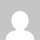Engineering often involves applying a consistent, structured approach to the solving of problems. A general problem-solving approach and method can be defined, although variations will be required for specific problems.

Problems must be approached methodically, applying an algorithm, or step-by-step procedure by which one arrives at a solution.

The problem-solving process for a computational problem can be outlined as follows:

1. Define the problem.
2. Create a mathematical model.
3. Develop a computational method for solving the problem.
4. Implement the computational method.
5. Test and assess the solution.

The boundaries between these steps can be blurred and for specific problems one or two of the steps may be more important than others. Nonetheless, having this approach and strategy in mind will help to focus our efforts as we solve problems

1. Problem Definition:

The first steps in problem solving include:

• Recognize and define the problem precisely by exploring it thoroughly (may be the most difficult step).
• Determine what question is to be answered and what output or results are to be produced.
• Determine what theoretical and experimental knowledge can be applied.
• Determine what input information or data is available

Many academic problems that you will be asked to solve have this step completed by the instructor.

For example, if your instructor ask you to solve a quadratic algebraic equation and provides you with all of the coefficients, the problem has been completely defined before it is given to you and little doubt remains about what the problem is.

If the problem is not well defined, considerable effort must be expended at the beginning in studying the problem, eliminating the things that are unimportant, and focusing on the root problem. Effort at this step pays great dividends by eliminating or reducing false trials, thereby shortening the time taken to complete later steps.

After defining the problem:

• Collect all data and information about the problem.
• Verify the accuracy of this data and information.
• Determine what information you must find: intermediate results or data may need to be

found before the required answer or results can be found.

2. Mathematical Model:

To create a mathematical model of the problem to be solved:

• Determine what fundamental principles are applicable.
• Draw sketches or block diagrams to better understand the problem.
• Define necessary variables and assign notation.
• Reduce the problem as originally stated into one expressed in purely mathematical terms.
• Apply mathematical expertise to extract the essentials from the underlying physical description of the problem.
• Simplify the problem only enough to allow the required information and results to be obtained.
• Identify and justify the assumptions and constraints inherent in this model.

3. Computational Method:

A computational method for solving the problem is to be developed, based on the mathematical model.

• Derive a set of equations that allow the calculation of the desired parameters and variables.
• Develop an algorithm, or step-by-step method of evaluating the equations involved in the solution.
• Describe the algorithm in mathematical terms and then implement as a computer program.
• Carefully review the proposed solution, with thought given to alternative approaches

4. Implementation of Computational Method:

Once a computational method has been identified, the next step is to carry out the method with a computer, whether human or silicon.

Some things to consider in this implementation:

• Assess the computational power needed, as an acceptable implementation may be hand calculation with a pocket calculator.
• If a computer program is required, a variety of programming languages, each with different properties, are available.
• A variety of computers, ranging from the most basic home computers to the fastest parallel supercomputers, are available.
• The ability to choose the proper combination of programming language and computer, and use them to create and execute a correct and efficient implementation of the method, requires both knowledge and experience. In your engineering degree program, you will be exposed to several programming languages and computers, providing you with some exposure to this issue.

The mathematical algorithm developed in the previous step must be translated into a computational algorithm and then implemented as a computer program.

The steps in the algorithm should first be outlined and then decomposed into smaller steps that can be translated into programming commands.

One of the strengths of Matlab is that its commands match very closely to the steps that are used to solve engineering problems; thus the process of determining the steps to solve the problem also determines the Matlab commands. Furthermore, Matlab includes an extensive toolbox of numerical analysis algorithms, so the programming effort often involves implementing the mathematical model, characterizing the input data, and applying the available numerical algorithms.

5. Test and Assess the Solution:

The final step is to test and assess the solution. In many aspects, assessment is the most open-ended and difficult of the five steps involved in solving computational problems.

The numerical solution must be checked carefully:

• A simple version of the problem should be hand checked.
• The program should be executed on obtained or computed test data for which the answer or solution is either known or which can be obtained by independent means, such as hand or calculator computation.
• Intermediate values should be compared with expected results and estimated variations.
• When values deviate from expected results more than was estimated, the source of the deviation should be determined and the program modified as needed.
• A “reality check” should be performed on the solution to determine if it makes sense.
• The assumptions made in creating the mathematical model of the problem should be checked against the solution.

Share it

updated on

by

## 2 responses to “Process of Solving Engineering Problems”

1.why is your post 5 years ago

2.pankaj raul

how to remove bearing after shrink fitting to reuse???

This site uses Akismet to reduce spam. Learn how your comment data is processed.